Share
Notifications

View all notifications
Books Shortlist
Your shortlist is empty

# Selina solutions for Class 10 Mathematics chapter 15 - Similarity (With Applications to Maps and Models)

## Selina ICSE Concise Mathematics for Class 10 (2018-2019)

Login
Create free account

Forgot password?

#### Selina Selina ICSE Concise Mathematics Class 10 (2018-2019)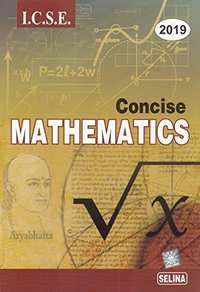## Chapter 15: Similarity (With Applications to Maps and Models)

15A15B15C

#### Chapter 15: Similarity (With Applications to Maps and Models) Exercise 15A solutions [Page 0]

true or false:

Two similar polygons are necessarily congruent.

true or false:

Two congruent polygons are necessarily similar.

true or false:

all equiangular triangles are similar.

true or false:

all isosceles triangles are similar.

true or false:

Two isosceles – right triangles are similar

true or false:

Two isosceles triangles are similar, if an angle of one is congruent to the corresponding angle of the other.

true or false:

The diagonals of a trapezium, divide each other into proportional segments.

In triangle ABC, DE is parallel to BC; where D and E are the points on AB and AC respectively.
Prove: ∆ADE ~ ∆ABC.
Also, find the length of DE, if AD = 12 cm, BD = 24 cm BC = 8 cm.

Given:
∠GHE = ∠DFE = 90 ,

DH = 8, DF = 12,
DG = 3x – 1 and DE = 4x + 2.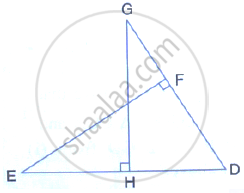Find: the lengths of segments DG and DE

D is a point on the side BC of triangle ABC such that angle ADC is equal to angle BAC. Prove
that: "CA"^2 = CB × CD

In the given figure, ∆ ABC and ∆ AMP are right angled at B and M respectively.
Given AC = 10 cm, AP = 15 cm and PM = 12 cm.
(i) Prove ∆ ABC ~ ∆ AMP
(ii) Find AB and BC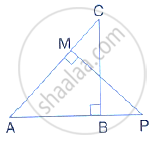E and F are the points in sides DC and AB respectively of parallelogram ABCD. If diagonal AC
and segment EF intersect at G; prove that:

Given: RS and PT are altitudes of ΔPQR. Prove that:
(i) Δ PQT ~ ΔQRS
(ii) PQ × QS = RQ × QT

Given: ABCD is a rhombus, DRP and CBR are straight lines.Prove that:
DP × CR = DC × PR

Given: FB = FD, AE ⊥ FD and FC ⊥ AD

Prove: ("FB")/("AD")=("BC")/("ED")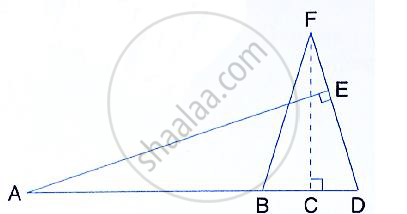In ∆ ABC, ∠B = 2 ∠C and the bisector of angle B meets CA at point D. Prove that:
(i) ∆ ABC and ∆ ABD are similar,
(ii) DC: AD = BC: AB

In ∆PQR, ∠Q = 90° and QM is perpendicular to PR. Prove that:
(i) PQ2 = PM × PR

(ii)QR2 = PR × MR

(iii) PQ2 + QR2 = PR2

In ∆ABC, right – angled at C, CD ⊥ AB.
Prove:
"CD"^2 = "AD"xx "DB"

In ∆ABC, ∠B = 90° and BD ⊥ AC.
(i) If CD = 10 cm and BD = 8 cm; find AD.
(ii) IF AC = 18 cm and AD = 6cm; find BD.
(iii) If AC = 9 cm and AB = 7cm; find AD.

In the figure, PQRS is a parallelogram with PQ = 16 cm and QR = 10cm, L is a point on PR
such that RL: LP = 2: 3. QL produced meets RS at M and PS produced at N.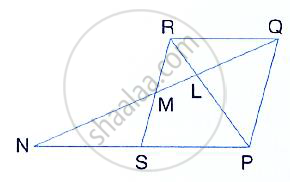Find the lengths of PN and RM.

In quadrilateral ABCD, diagonals AC and BD intersect at point E such that
AE: EC = BE: ED
Show that ABCD is a parallelogram.

Given: AB || DE and BC || EF. Prove that:

(AD)/(DG)=(CF)/(FG)

 ∆"DFG"  ~ ∆"ACG"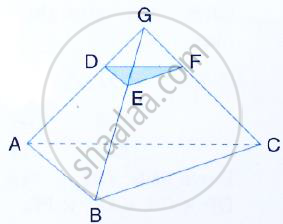In triangle ABC, AD is perpendicular to side BC and AD2 = BD × DC.
Show that angle BAC = 90°

In the given figure, AB ∥ EF ∥ DC ; AB = 67.5 cm, DC = 40.5 cm and AE = 52.5 cm.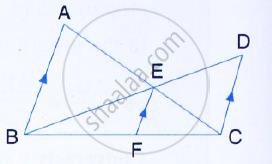(i) Name the three pairs of similar triangles.
(ii) Find the lengths of EC and EF.

In the given figure, QR is parallel to AB and DR is parallel to AB and DR is parallel to QB.
Prove that: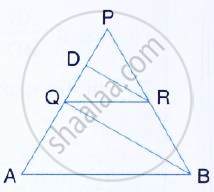PQ^2= PD × PA

Through the mid-point M of the side CD of a parallelogram ABCD, the line BM is drawn
intersecting diagonal AC in L and AD produced in E. Prove that: EL = 2 BL.

In the figure, given below, P is a point on AB such that AP : PB = 4 : 3. PQ is parallel to AC.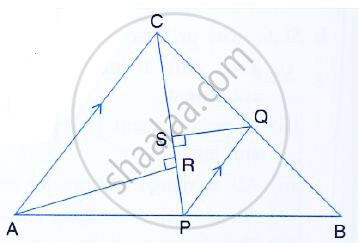(i) Calculate the ratio PQ : AC, giving reason for your answer.
(ii) In triangle ARC, ∠ARC = 90°. Given QS = 6cm, calculate the length of AR.

In the right-angled triangle QPR, PM is an altitude.
Given that QR = 8cm and MQ = 3.5 cm, calculate the value of PR.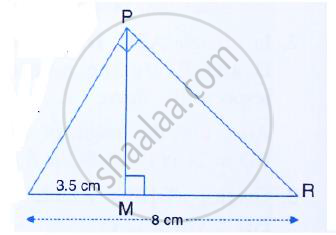In the figure, given below, the medians BD and CE of a triangle ABC meet at G. Prove that:
(i) Δ EGD ~ ΔCGB and
(ii) BG = 2 GD from (i) above.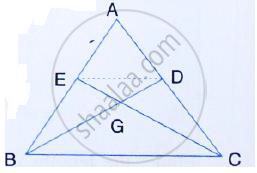#### Chapter 15: Similarity (With Applications to Maps and Models) Exercise 15B solutions [Page 0]

The ratio between the corresponding sides of two similar triangles is 2 is to 5. Find the ratio
between the areas of these triangles.

Area of two similar triangles are 98 sq.cm and 128 sq.cm. Find the ratio between the lengths
of their corresponding sides.

A line PQ is drawn paral el to the base BC of ∆ABC which meets sides AB and AC at points P
and Q respectively. If AP= 1/3PB; find the value of:

1) "Area of ΔABC"/"Area of ΔAPQ"

2)"Area of ΔAPQ"/"Area of trapeziumPBCQ"

The perimeter of two similar triangles are 30 cm and 24 cm. If one side of the first triangle is 12 cm, determine the corresponding side of the second triangle.

In the given figure, AX : XB = 3: 5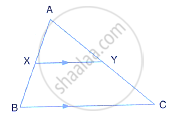(i) the length of BC, if the length of XY is 18 cm.

(ii) the ratio between the areas of trapezium XBCY and triangle ABC.

ABC is a triangle. PQ is a line segment intersecting AB in P and AC in Q such that PQ ∥ BC and divides triangle ABC into two parts equal in area. Find the value of ratio BP : AB.

In the given triangle PQR, LM is parallel to QR and PM : MR = 3: 4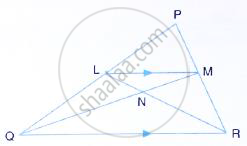Calculate the value of ratio:

1) ("PL")/("PQ") "and then" ("LM")/("QR")

2) "Area of Δ LMN"/"Area of Δ MNR"

3) "Area of Δ LQM"/"Area of Δ LQN"

The given diagram shows two isosceles triangles which are similar also. In the given diagram,
PQ and BC are not parallel; PC = 4, AQ = 3, QB = 12, BC = 15 and AP = PQ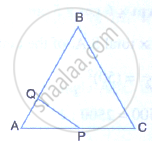Calculate:
(i) the length of AP,
(ii) the ratio of the areas of triangle APQ and triangle ABC.

In the figure, given below, ABCD is a parallelogram. P is a point on BC such that BP : PC = 1:
2. DP produced meets AB produces at Q. Given the area of triangle CPQ = 20 cm2.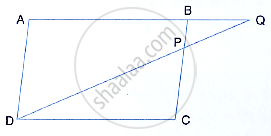Calculate:
(i) area of triangle CDP,
(ii) area of parallelogram ABCD.

In the given figure, BC is parallel to DE. Area of triangle ABC = 25 cm2, Area of trapezium BCED = 24 cm2 and DE = 14cm.
Calculate the length of BC. Also, find the area of triangle BCD.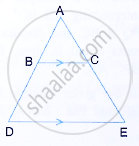The given figure shows a trapezium in which AB is parallel to DC and diagonals AC and BD
intersect at point P. If AP : CP = 3: 5,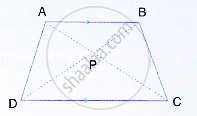Find:
(1) ∆APB : ∆CPB (2 ) ∆DPC : ∆APB
(3) ∆ADP : ∆APB  (iv) ∆APB : ∆ADB

On a map, drawn to a scale of 1 : 250000, a triangular plot PQR of land has the following measurements :

PQ = 3cm, QR = 4 cm and angles PQR = 90°

(i) the actual lengths of QR and PR in kilometer.
(ii) the actual area of the plot in sq . km.

A model of a ship if made to a scale of 1 : 200.
(i) Thelength of the model is 4 m; calculate the length of the ship.

(ii) The area of the deck of the ship is 160000 m2; find the area of the deck of the model.
(iii) The volume of the model is 200 litres; calculate the volume of the ship in m3.

In the given figure, ABC is a triangle. DE is parallel to BC and ("AD")/("DB")=3/2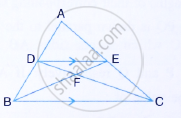(i) Determine the ratios ("AD")/("AB") and ("DE")/("BC")

(ii) Prove that ∆DEF is similar to ∆CBF Hence, find ("EF")/("FB").

(iii) What is the ratio of the areas of ∆DEF and ∆BFC.

In the given figure, ∠B = ∠E, ∠ACD = ∠BCE, AB = 10.4cm and DE = 7.8 cm. Find the ratio
between areas of the ∆ABC and ∆ DEC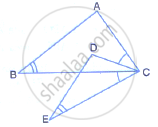Triangle ABC is an isosceles triangle in which AB = AC = 13 cm and BC = 10 cm. AD is
perpendicular to BC. If CE = 8 cm and EF ⊥ AB, find:

i)"area of ADC"/"area of FEB"       ii)"area of ΔAFEB"/"area of ΔABC"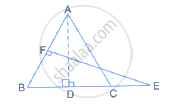An aeroplane is 30 m long and its model is 15 cm long. If the total outer surface area of the model is 150 cm2, find the cost of painting the outer surface of the aeroplane at the rate of Rs.120 per sq. m. Given that 50 sq. m of the surface of the aeroplane is left for windows.

#### Chapter 15: Similarity (With Applications to Maps and Models) Exercise 15C solutions [Page 0]

In the given figure, lines l, m and n are such that ls ∥ m ∥ n. Prove that:

(AB)/(BC)=(PQ)/(QR)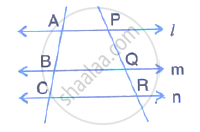In the given triangle P, Q and R are the mid points of sides AB, BC and AC respectively. Prove that triangle PQR is similar to triangle ABC.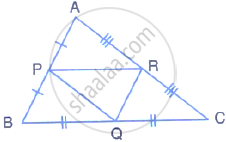In the following figure, AD and CE are medians of ΔABC. DF is drawn parallel to CE. Prove that :
(i) EF = FB,
(ii) AG : GD = 2 : 1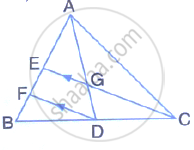In the given figure, triangle ABC is similar to  triangle PQR. AM and PN are altitudes whereas AX and PY are medians.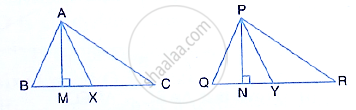prove  that

("AM")/("PN")=("AX")/("PY")

The two similar triangles are equal in area. Prove that the triangles are congruent.

The ratio between the altitudes of two similar triangles is 3 : 5; write the ratio between their:
(i) medians (ii) perimeters (iii) areas

The ratio between the areas of two similar triangles is 16 : 25, Find the ratio between their:
(i) perimeters (ii) altitudes (iii) medians

The following figure shows a triangle PQR in which XY is parallel to QR. If PX : XQ = 1 : 3 and QR = 9 cm. find the length of XY.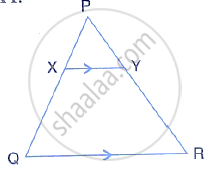Further, if the area of Δ PXY =x cm2 ; find, in terms of x the area of:

(i) triangle PQR (ii) trapezium XQRY

In the following figure, AB, CD and EF are parallel lines. AB = 6cm, CD = y cm, EF = 10 cm, AC = 4 cm and CF = x cm. Calculate x and y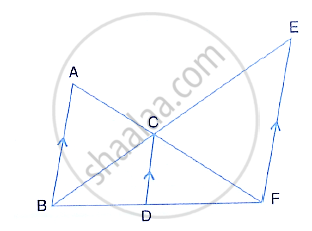On a map, drawn to a scale of 1 : 20000, a rectangular plot of land ABCD has AB = 24cm and BC = 32 cm. Calculate:

(i) the diagonal distance of the plot in kilometer

(ii) the area of the plot in sq.km

The dimensions of the model of a multistoreyed building are 1 m by 60 cm by 1.20 m. if the scale factor is 1 : 50, find the actual dimensions of the building.

Also find:

(i) the floor area of a room of the building, if the floor area of the corresponding room in the model is 50 sq. cm

(ii) the space (volume) inside a room of the model, if the space inside the corresponding room of the building is 90 m^3

In a triangle PQR, L and M are two points on the base QR, such that ∠LPQ = ∠QRP and ∠RPM

= ∠RQP. Prove that:

(i) ΔPQL and ΔRMP
(ii) QL  xx  RM = P L  ×    PM
(iii)  PQ^2  =   QR  × QL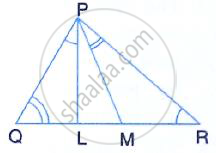In ΔABC, ∠ACB = 90° and CD ⊥ AB.

Prove that : (BC^2)/(AC^2)=(BD)/(AD)

A triangle ABC with AB = 3 cm, BC = 6 cm and AC = 4 cm is enlarged to ΔDEF such that the longest side of ΔDEF = 9 cm. Find the scale factor and hence, the lengths of the other sides of ΔDEF.

Two isosceles triangles have equal vertical angles. Show that the triangles are similar.If the ratio between the areas of these two triangles is 16 : 25, find the ratio between their corresponding altitudes.

In ΔABC, AP : PB = 2 : 3. PO is parallel to BC and is extended to Q so that CQ is parallel to BA.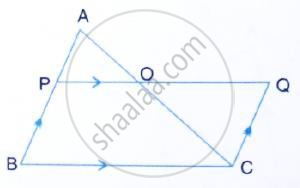Find:

(i) area ΔAPO : area Δ ABC.

(ii) area ΔAPO : area Δ CQO.

The following figure shows a triangle ABC in which AD and BE are perpendiculars to BC and AC respectively.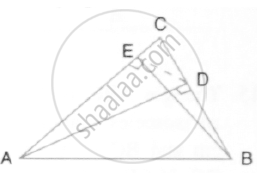Show that;

(i) ΔADC ~ ΔBEC

(ii) CA × CE = CB × CD

(iii) ΔABC ~ ΔDEC

(iv) CD × AB = CA × DE

In the give figure, ABC is a triangle with ∠EDB = ∠ACB. Prove that ΔABC ~ ΔEBD. If BE =6 cm, EC = 4cm, BD = 5cm and area of ΔBED = 9 cm2. Calculate the:

(i) length of AB]

(ii) area of Δ ABC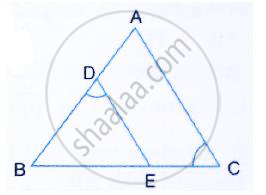In the given figure, ABC is a right angled triangle with ∠BAC = 90°.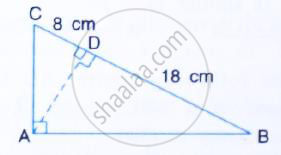(i) Prove ΔADB ~ ΔCDA.
(ii) If BD = 18 cm and CD = 8cm, find AD.
(iii) Find the ratio of the area of ΔADB is to area of ΔCDA.

## Chapter 15: Similarity (With Applications to Maps and Models)

15A15B15C

#### Selina Selina ICSE Concise Mathematics Class 10 (2018-2019)## Selina solutions for Class 10 Mathematics chapter 15 - Similarity (With Applications to Maps and Models)

Selina solutions for Class 10 Maths chapter 15 (Similarity (With Applications to Maps and Models)) include all questions with solution and detail explanation. This will clear students doubts about any question and improve application skills while preparing for board exams. The detailed, step-by-step solutions will help you understand the concepts better and clear your confusions, if any. Shaalaa.com has the CISCE Selina ICSE Concise Mathematics for Class 10 (2018-2019) solutions in a manner that help students grasp basic concepts better and faster.

Further, we at Shaalaa.com are providing such solutions so that students can prepare for written exams. Selina textbook solutions can be a core help for self-study and acts as a perfect self-help guidance for students.

Concepts covered in Class 10 Mathematics chapter 15 Similarity (With Applications to Maps and Models) are Areas of Similar Triangles Are Proportional to the Squares on Corresponding Sides, Axioms of Similarity of Triangles, Similarity Triangle Theorem, Similarity of Triangles.

Using Selina Class 10 solutions Similarity (With Applications to Maps and Models) exercise by students are an easy way to prepare for the exams, as they involve solutions arranged chapter-wise also page wise. The questions involved in Selina Solutions are important questions that can be asked in the final exam. Maximum students of CISCE Class 10 prefer Selina Textbook Solutions to score more in exam.

Get the free view of chapter 15 Similarity (With Applications to Maps and Models) Class 10 extra questions for Maths and can use Shaalaa.com to keep it handy for your exam preparation

S